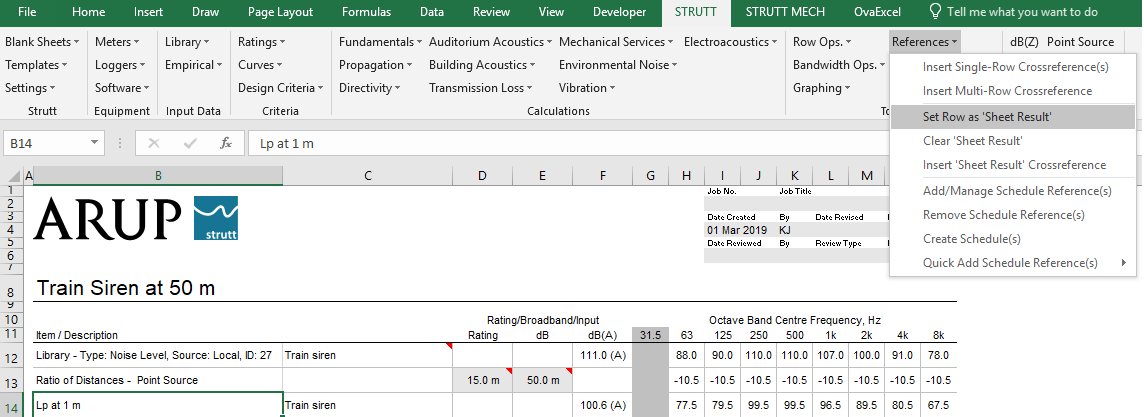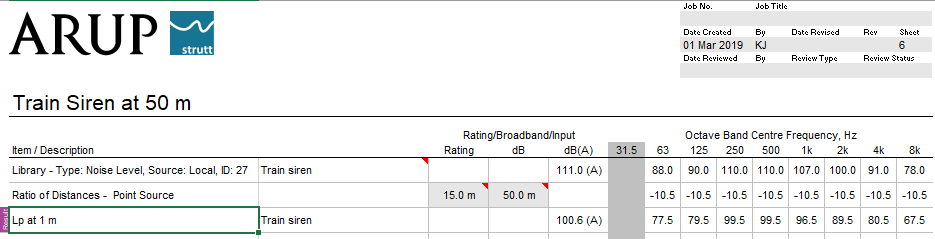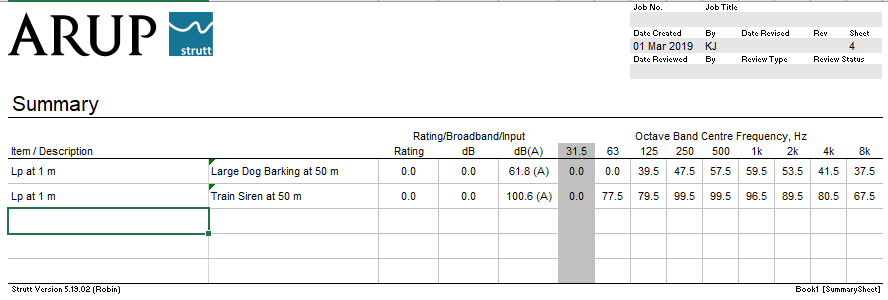﻿ Summary Page / Sheet Result### How To "Strutt" Series

How To Use the Summary Page / Sheet Result Feature    1/1, 1/3

The Sheet Result feature in Strutt provides an easy way of referencing the row containing the result of a calculation on a worksheet.

Go to Tools → References → Set Row As "Sheet Result" (Figure 1). You will be prompted to select the row in your worksheet that contains the result of your calculation. A label will be added to column A of the Result row (Figure 2).Figure 1: Select and label the result rowFigure 2: Result row is labelled in column A

If you have multiple worksheets, you can summarise the results in a Summary sheet (accessed via Strutt → Blank Sheets → Summary). Each Result row in the workbook will be listed in the Summary sheet (Figure 3).Figure 3: Summary sheet lists results of each worksheet in the workbook

If you label a different row as the result of a sheet, the Summary sheet will automatically update.

Similarly, you can also cross-reference a sheet's Result row on another sheet via Tools → References → Insert "Sheet Result" Crossreference.

You can clear the results label on a sheet via Tools → References → Clear "Sheet Result".

Happy Strutting!